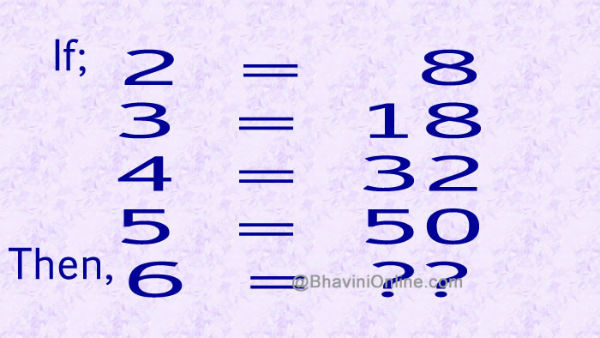# Whatsapp Number Riddle: If 2 = 8, Then 6 = ?

If you like solving Mathematical Riddles, you will enjoy solving this.

You have to find the value of ?? by looking at the sequence;

If

2 = 8

3 = 18

4 = 32

5 = 50

6 = ??So were you able to solve the riddle? Leave your answers in the comment section below.